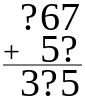Want to share your content on R-bloggers? click here if you have a blog, or here if you don't.The `aggregate()` function subsets dataframes, and time series data, then computes summary statistics. The structure of the `aggregate()` function is `aggregate(x, by, FUN)`.

Answers to the exercises are available here.

Exercise 1
Aggregate the “`airquality`” data by “`airquality\$Month`“, returning means on each of the numeric variables. Also, remove “`NA`” values.

Exercise 2
Aggregate the “`airquality`” data by the variable “`Day`“, remove “`NA`” values, and return means on each of the numeric variables.

Exercise 3
Aggregate “`airquality\$Solar.R`” by “`Month`“, returning means of “`Solar.R`“. The header of column 1 should be “`Month`“. Remove “`not available`” values.

Exercise 4
Apply the standard deviation function to the data aggregation from Exercise 3.

Exercise 5
The structure of the `aggregate()` formula interface is `aggregate(formula, data, FUN)`.

The structure of the formula is `y ~ x`. The “`y`” variables are numeric data. The “`x`” variables, usually factors, are grouping variables, that subset the “`y`” variables.

`aggregate.formula` allows for one-to-one, one-to-many, many-to-one, and many-to-many aggregation.

Therefore, use `aggregate.formula` for a one-to-one aggregation of “`airquality`” by the mean of “`Ozone`” to the grouping variable “`Day`“.

Exercise 6
Use `aggregate.formula` for a many-to-one aggregation of “`airquality`” by the mean of “`Solar.R`” and “`Ozone`” by grouping variable, “`Month`“.

Exercise 7
Dot notation can replace the “`y`” or “`x`” variables in `aggregate.formula`. Therefore, use “`.`” dot notation to find the means of the numeric variables in `airquality`“, with the grouping variable of “`Month`“.

Exercise 8
Use dot notation to find the means of the “`airquality`” variables, with the grouping variables of “`Day`” and “`Month`“. Display only the first 6 resulting observations.

Exercise 9
Use dot notation to find the means of “`Temp`“, with the remaining “`airquality`” variables as grouping variables.

Exercise 10
`aggregate.ts` is the time series method for `aggregate()`.

Using `R`‘s built-in time series dataset, “`AirPassengers`“, compute the average annual standard deviation.

Image by Averater (Own work) [CC BY-SA 3.0], via Wikimedia Commons.